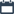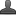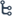## Tuesday, October 13, 2020

•October 13, 2020
••,
•No comments

## Introduction to Real numbers (9th class )Chapter-1,Notes,Definition,concept,important points

Real numbers

Hi friends and my dear students! In this post, I have covered Andhra Pradesh class-9 Introduction to Real numbers (9th class ) chapter-1 important points After Reading Mathematics Real numbers Definition Notes With important points, please do share it with your friends. You can Learn Maths for All Classes here.
Natural Numbers: The counting numbers 1,2,3,4,5,6.....are called  Natural numbers. It is represented by 'N'  .N= (1, 2, 3, 4, 5, 6 ... .}The smallest a natural number is 1 and the greatest natural number does not exist

Whole Numbers: Natural numbers including zero are called whole numbers. It is Represented by W. W= {0,1,2,3,4,5,6,... } The least whole number is 0 and the greatest whole number does not exist.

Integers: The set containing the positive numbers, the negative numbers together with zero is called the set of integers.

Whole numbers including negative numbers are called Integers. It is represented by Z. The set of integers is represented by Z.

Z = {... - 4, -3, -2, -1, 0,1,2,3,4.....}

Note:

1{ 1.2,3,............} is called  Set of positive integers.

2. { -1,-2,-3 ,...............} is called  Set of negative integers. ,

3. Zero is considered neither positive nor negative.

4. In the number line all negative integers are on the left side of zero, all positive integers are on the right side of zero.

5. Set of positive integers and zero considered as Non – negative integers. {0,1,2,3,.......} is set of non – negative integers.

6. Set of negative integers and zero considered as Non - positive integers. (0,-1,-2,....} is set of non - positive integers.

7. The differences between any two consecutive integers is 1.

8. The absolute value of an integer is the numerical value of the integer regardless of its sign.

*Numbers of the form P/q where p and q are integers and q≠0 are called rational numbers, represented by 'Q'.

*There are infinitely many rational numbers between any two integers

E.g. 3 < 29 /6, 20/6, 21/6, 22/6, 23/6 ....... < 4

*There are infinitely many rational numbers between any two rational numbers.

E.g.  3/4< 29 /8, 71/ 16, 81/ 14........<13/2

*To find the decimal representation of a rational number we divide the numerator of a rational number by its denominator.

E.g. : The decimal representation of 5/6

7/6=1.1666....

Also Check

Introduction to Knowing Our Numbers Key Points

Introduction to integers (7th class)

Trigonometry Do This & Try this solution

Introduction To Trigonometry

SSC (10th class) Trigonometry Exercise - 11.1 Solution

SSC(10th class) Trigonometry Exercise - 11.1 Solutions

*Every rational number can be expressed as a terminating decimal or as non-terminating repeating decimal. Conversely, every terminating decimal or non- terminating recurring decimal can be expressed as a rational number 7-29

=361/495

* A rational number whose denominator consists of only 2's or 5's or a combination of 2's and 5's can be expressed as a terminating decimal. Can be expressed as a terminating decimal.

EG: 13/32   can be expressed as a terminating decimal (32 = 2 x 2 x 2 x 2 x 2)

7/125 can be expressed as a terminating decimal (125 =5 x 5 x 5)

24 /40 Can be expressed as a terminating decimal (40 = 2 x 2x2x5)

*Numbers which can't be written in the form where p and q are integers and q ≠0.are called irrational numbers. E.g: V2, V3, V5...etc.

*The decimal form of an irrational number is neither terminating nor recurring decimal.

*If 'n' is a natural number which is not a perfect square, then Vn is always an irrational number. E.g. : 2, 3, 5, 7, 8, . etc., are not perfect squares.

V2, V3, V5, V7 and V8 are irrational numbers.

*The collection of rational numbers together with irrational numbers is called set of Real numbers. R = QUS

* If a and b are two positive rational numbers such that ab is not a perfect square, then Vab is an irrational number between 'a' and 'b'.

E.g: Consider any two rational numbers 7 and 4.

7 x 4 = 28 is not a perfect square; then 28 lies between 4 and 7.

i.e.4 < V28 < 7

* If 'a' is a rational number and 'b' is any irrational number then a + b, a - b, a. b or a/b is an irrational number.

E.g.: Consider 8 and V 7 then 8+V7, 8 - V7, 8/V7 and are all irrational numbers

* If the product of any two irrational numbers is a rational number, then they are said to be the rationalising factor of each other.

E.g.  : Consider any two irrational number 7/ V 5 and 6/ V5.

7V 5 x 5 V 5  =    7 x 5 x5 =   175    a rational number.

Also 73 x 3 =   219       - a rational number.

5 V 4 x V 4 = 20           - a rational number.

So  The rationalising factor of an irrational number is not unique.

*The general form of rationalizing factor (R.F.) of (a _+ vb) is (a +vb). They are called conjugates to each other.

*Laws of Exponents:

1. am . an =  a m+n

2. (am)n= amn

am /bn = a m-n       if m > n

=1                     if m=n

=1/ an-m               if m < n

*1/ an=a-n

*am  . bn= (ab)n

*a0   = 1

*(Va+b)=a+2Vab+b2

*Va×Vb =Vab

*(Va+b)(Va-b)=a-b2

Where a, b are rationales and m, n are integers.

* Let a, b be any two rational numbers such that a = bn then b = nVa   = a1/n Here 'b' is called nth root of a.

* Let 'a' be a positive number and n > 1 then n

i.e, nth root of a is called a surd.